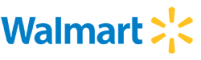New update is available. Click here to update.
Topics

# Longest Bracket

Moderate0/80
Average time to solve is 20m## Problem statement

You are given a string S, consisting of only '(' and ')' characters. You need to find the length of the longest substring which is a regular bracket sequence and also find the count of such substrings with the same length.

Note:

``````A bracket sequence is called regular, if parenthesis in the given expression is balanced.  For example '()()', '(())' are the regular string but '((()' is not a regular parenthesis string.

If no such substring exists, print "0 1" (without quotes).
``````
Detailed explanation ( Input/output format, Notes, Images )
Constraints :
``````1 <= T <= 5
1 <= N <= 10 ^ 4

Time Limit : 1 sec.
``````

Note :

``````You don’t need to print anything, it has already been taken care of. Just implement the given function.
``````

##### Sample Input 1:

``````2
)((())))(()())
))(
``````
Sample Output 1 :
``````6 2
0 1
``````
Explanation for Sample Input 1 :
``````Test Case 1:
The longest balanced parentheses substring starts from index 1 and ends at index 6 making the count of longest substring 6 and there is another balanced substring of length = 6 starting from index 8 and ending at index 13.

Therefore, the total count of balanced parentheses substring is 2. Hence the output is “6 2”.

Test Case 2:
There is no such balanced parentheses substring exists in the input string so the length of the longest balanced parentheses substring is 0 and 1 will be the count of such substring.
``````

##### Sample Input 2 :

``````2
)()()()()()()
(())(
``````
Sample Output 2 :
``````12 1
4 1
``````
Explanation for Sample Input 1 :
``````Test Case 1:
The longest balanced parentheses substring starts from index 2 and ends at index 13 with length  = 12 and total count 1.

Test Case 2:
The longest balanced parentheses substring starts from index 1 and ends at index 4 with length  = 4 and total count 1.
``````Console